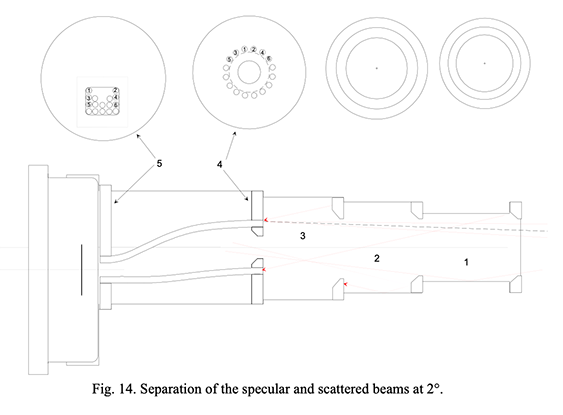OPO - Monitoring the reflectivity and scattering of telescope's coatings
Notes

Content:
Application note #4
Basics of straylight definition and measurement

# Application note #4

## Basics of straylight definition and measurement

Author: Daniel Malaise, Dr Sc
First issue: September 1996
Last review: August 2020

This document is the sole property of OPO and, except to learn about our work, may not be used without our written consent.

### Introduction

This note had been written to introduce the user of Iris scatterometer to the basic of stray light definition and measurements. The content fully applies to the newest CT7 and CT7a instruments.

There is some theory in the note: just what is necessary in order to understand the meaning of scattering. The main part of the note deals with practical matters related to the measurement.

Scattering is the ‘poor child’ in the field of optics, specially in Europe. It is however used in many domains. By nature, it is opposed to the phenomenon of specular reflection , that part of the reflected light that forms an image.

Scattering is used either positively when specular reflection is not wished: one uses paints or coatings that diffuse a maximum. This is the case of screens and of optical mounts.

It is also used negatively when considering optical surfaces that are used for specular reflection or refraction: in this case, all kinds of defects (microrugosity, scratches, dust, pollution, dirt etc.) produce scattering.

The latter can be used to measure the severity of those defects.

#### Solid angle

Optical radiometric computations utilize the notion of solid angle (Fig. 1).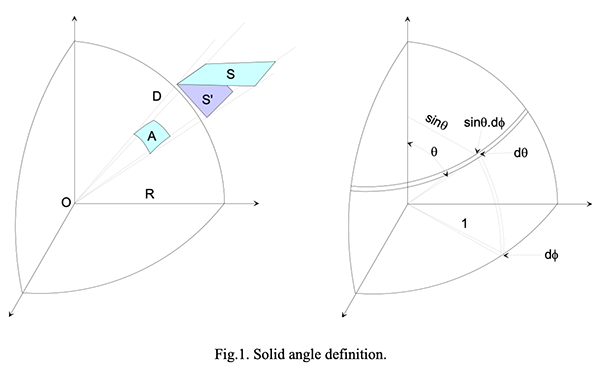Let us consider a sphere of radius R centered on the point O ; we define the solid angle Ω subtended by a surface S which does not contain O, as the region comprised between the point O and the straight line generator through O and leaning on the surface contour. Its numerical value expressed in steradians (str), equals the area A of the surface S projected on the sphere divided by the square of the radius of the sphere, i.e. Ω = A/R 2 . As the area of the entire sphere has the value 4πR 2 , the solid angle covering the whole space around a point has a value of 4π str, and the space around a point of a plane limited to one side of the plane has the value 2π str. Practically, if D 2 >>S, the solid angle subtended by this surface seen from a point O has a value S’/D 2 where S’ is the projection of S upon a plane perpendicular to the direction OS and D the distance from O to this plane. If r is the linear characteristic dimension of S, S’ has the same area as the projection of S on the sphere of radius D with an error smaller than 0.25π.D 2 . (r/D) 4 . For instance a detector of 1 mm 2 at 100 mm from a laser diode will see the source with a solid angle of 1/(100 2 )=10 -4 str with an error of .0008%; if the distance is shortened to 25 mm, the solid angle 1/(25 2 )=0.0016 str will still be correct within 0.13%.

#### Beam etendue or throughput

The computation of the transfer of radiative power from one surface to another is conditioned by the radiometric properties of the two surfaces and of the medium between the surfaces and by the geometry of the system ; it is practical to separate the geometrical factor called the beam spread (Fig.2.).

Let us consider two very small surface elements dA1 and dA2 (the d is there to indicate that the surfaces are extremely small and plane) separated by a distance R<; the normals N1 and N2 to these surfaces are indicated on the figure. The vector radius R makes respectively the angles θ1 and θ2 with these normals. The beam etendue is the quantity:

d 2 E = da 1 .cos(θ 1 ).da 2 .cos(θ 2 )/R 2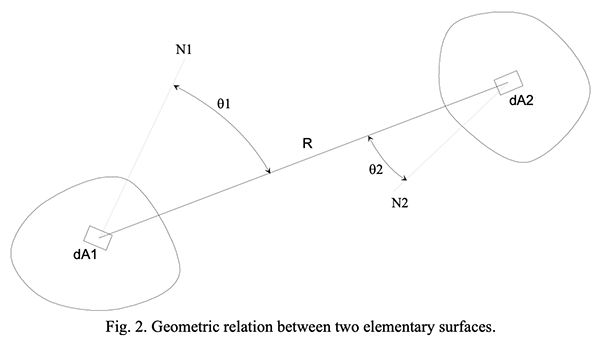But we have seen that da.cos(θ)/R 2 = dΩ ; hence one could also write:

d 2 E = da 1 .cos(θ 1 ).dΩ 2 = da 2 .cos(θ 2 ).dΩ 1

Radiometry deals with the energy transported by radiation; the latter is designated by the symbol Q and is measured in Joules (J). The energy by unit of time or flux of energy is the power P expressed in Watts (W) equivalent to one Joule per second.

This radiant energy is produced by sources and received or measured by detectors; these two components are characterized geometrically by their useful area. In nature, there is no such thing as a “point”, but there are emitting (or receiving) volumes. These will not be considered here; they can always be treated by enclosing them completely in a surface and applying the surface formalism.

The quantity of energy through a unit area per unit time is the flux density dP/da or power per unit area; the flux density is called differently when the energy leaves the surface ( exitance M) or arrives on it ( incidence H); in both cases it is the energy going through the surface in all directions.

The intensity is the power or energy flux leaving a source per unit solid angle; it comprises the power emitted by the entire source (whole surface), hence, one can talk of the intensity of a light bulb or of a discharge tube. The intensity might vary with the direction from the source.

The radiance of a source is the power emitted per unit area projected normally to the direction of observation and per unit solid angle.

All these quantities can be defined spectrally, that is when the radiation is not approximately monochromatic, one consider the quantity per unit wavelength (usually µm -1 , also nm -1 ) and one writes Q λ P λ etc. They are summarized in the following table and in Fig.3.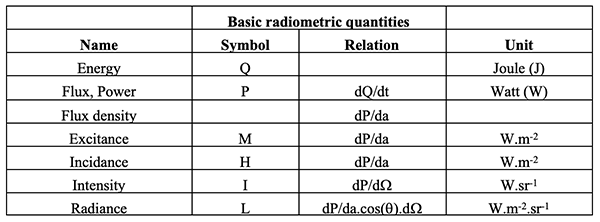Notice that the radiance is also the power per unit etendue and one could also write :

d 2 P = L.da.cos(θ).dΩ = L.dE = I.dΩ

for the power going through the two surfaces when there is no loss (or production) of energy between the surfaces (no absorption, no sources).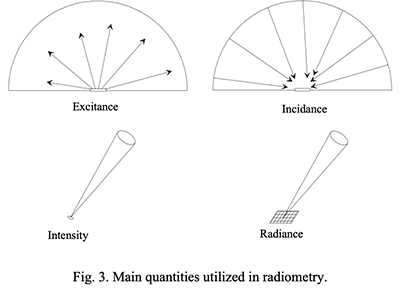#### Radiation transfer from one surface to another.

For computing the power transfer between two surfaces we need only to know the etendue defined by the two surfaces, the radiance of the emitting surface and the absorption of the receiving surface; the latter is described by an absorption coefficient ε = 1-a where a is the albedo or the total proportion of flux reflected back by the surface either by specular reflection or by reflective scattering .

The total power absorbed by the detector is described by the differential relation dP = L.ε.dE that has to be integrated over the etendue of the beam or practically over the area of the detector and the solid angle of the source surface as seen from the detector. Note that the etendue being symmetric with respect to the two surfaces, one could also integrate over the surface of the source and over the solid angle of the detector as seen from the source. If the medium between the two surface has a bulk transmission of T, the relation is simply dP = L.ε.T.δE.

Note that usually the coefficient ε is included in the transfer function of the detector (A/Watt) which transform the optical signal in amperes per incident watt (and not per absorbed watt). In this case the absorption coefficient should not be repeated explicitly in the formula.

The coefficients L, ε and T depend in general on the wavelength and on the incidence angle. When there is no dependence on the angle, the surface is said to be “lambertian”. When there is no dependence with the wavelength, the surface or the medium is said to be grey.

Diffusing surfaces (ground glass, velvet paint, lamp filament) show a more or less lambertian character; when one wishes to produce a true lambertian beam, one uses different special tricks: a few paint or materials are very close to lambertian (sodium salicylate, spectralon of lambdasphere); a hollow sphere painted inside with a lambertian paint and observed through a small hole is extremely close to a lambertian source and is uniform. All these bodies, when lit by any beam of light re-emit a lambertian beam. Hence, if one sends a uniform laser beam of power P on a lambertian surface of area a and albedo A, the total scattered power of the surface is: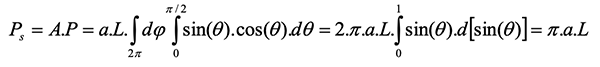and the constant radiance of the surface is: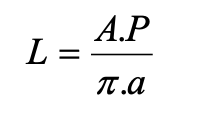If one observes the whole lit surface from a direction at an angle θ with the normal to the surface, the observed intensity will be:

I(θ) = P.A.cos(θ)/π

The power received by a detector of area S at a distance R (S<<R 2 ) in the direction θ will be:

W = I.T.Ω = P.A.ε.T.cos(θ).S/π.R 2

since Ω = S/R2 and T is the transmission of the medium separating the surfaces. Here, the detector is assumed to be perpendicular to the measured beam (fig.5a). In this relation, all the factors are constant with the direction of observation except for the cosine factor; if one plots the power versus the angle in a polar diagram, one obtains the well-known lambertian diagram fig 4a. Note that this diagram is obtained only if the detector sees all the lit surface; if, the surface were illuminated uniformly with collimated light (Fig.4b), the diagram of the power measured by the detector would be a constant.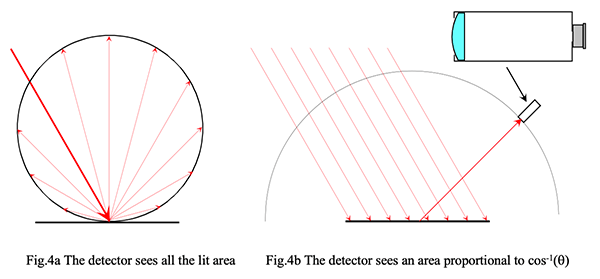The situation of Fig.4a is the one encountered in Iris , CT7 , CT7a and in most scatterometers. The situation of Fig.4b is also quite common; the lens projects the surface of the detector onto the emitting surface and the actual surface sending flux to the detector is proportional to 1/cos(θ). This situation happens when one observes with a telescope or with the naked eye: the brightness of a lambertian surface uniformly lit does not change with the angle of observation. This explains for instance why the moon is seen as a plain disk and not as a ball : it diffuses light in a roughly lambertian way.

When the detector is parallel to the emitting surface (Fig.5b) we are in the situation of a detector in the focal plane of a lens; then, Ω=S.cos(θ)/R 2 and the power will be

W = I.TΩ = P.a.ε.T.cos 2 (θ).S/π.R 2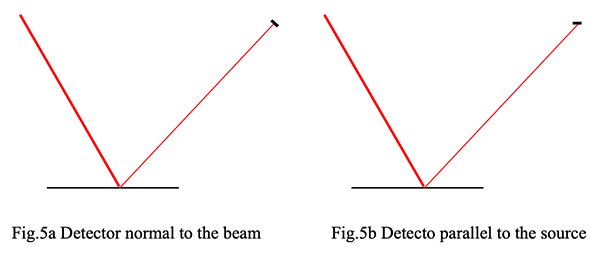#### Bulk absorption

An absorbing medium is characterized by its linear absorption coefficient defined by the differential relation:

dP = - κ.P.dx

with

dx → 0

which simply tells us that the loss of power from the beam by crossing a very small thickness dx of the medium is proportional to the incident power P and to the crossed thickness; since absorption will decrease the power of the beam the proportional coefficient is negative and is written –κ. If the medium is homogeneous, κ is a constant and the differential equation can be integrated, yielding: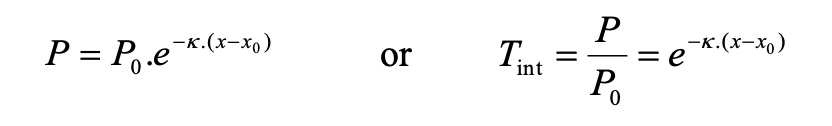where P is the energy flux at x, P 0 the energy flux at x 0 and T the bulk (or internal) transmission for a thickness of x-x 0 along the beam.

When considering a glass plate of thickness d crossed at an (refracted) angle θ by a parallel beam, the external transmission is the ratio of the flux after and before crossing the plate.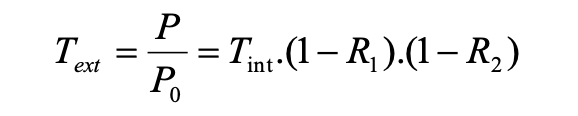where R 1 and R 2 are the Fresnel reflectivities at the entering and leaving the plate, and T int = e -κ.d/cos(θ) is the bulk or internal transmission of the glass.

### Scattering measurement

#### What is the usual way of expressing the scattering of an optical surface?

There are basically two related ways: angle-resolved scattering or Bidirectional Scattering Distribution Function ( BSDF ), and Total Integrated Scattering ( TIS ). The latter is the integration of the former over the whole sphere divided by the albedo.The distribution function is mostly utilized in its simplified form limited to the incidence plane (φ=0):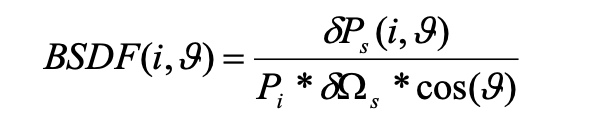In this expression (another distribution function is sometimes used; it is known as the angle resolved scattering function (ARS); it is simply the BSDF multiplied by cos(θ)), i is the incidence angle of the source beam (from the normal to the scattering surface), θ is the scattering direction (from the normal), P i is the power in the incident beam, δP s is the power in the scattered beam inside the solid angle δΩ s around the direction θ . It is assumed that the whole illuminated surface is seen by the scattering detector or that the powers are expressed per unit area of the scatterer. The BSDF is expressed in steradians -1 . These measurements are well known and documented in optics (see Jean M. Bennet and Lars Marrsson, Surface roughness and scattering, OSA, Washington D.C. and John C. Stover, Optical Scattering, SPIE Opt. Eng. Press).

One can rearrange the BSDF definition equation to show its relation to the radiometric quantity "radiance" by replacing the total incoming power by the power per surface unit δa: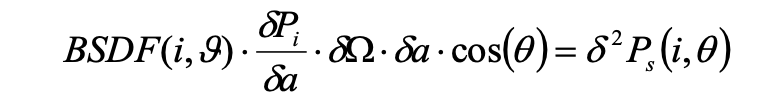one sees, by comparing with the definitions on p. 3, that the radiance is :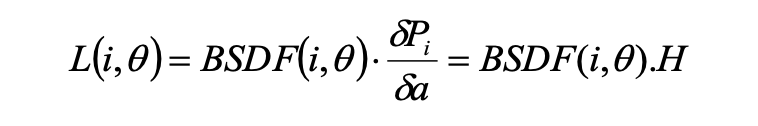(the radiance is what remains constant in a "lambertian" source).

The BSDF is a monotonous decreasing function which covers several orders of magnitudes when going from small angles with respect to the specular direction (the direction where the beams proceeds when there is no scattering) to large angles. When the scattering is measured on the side of the transmitted beam, one calls the function the BTDF, and when measured on the side of the reflected beam, it is called the BRDF (so that the BSDF of a transparent surface is the junction of its BTDF and its BRDF).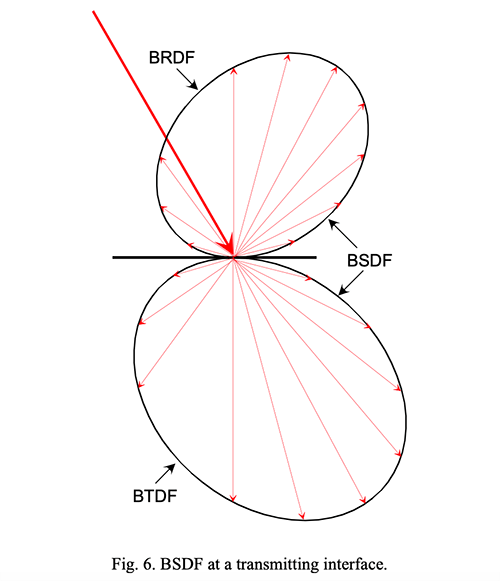#### How do we measure the scattering properties of a surface?

The scattering properties of a surface is measured by a scatterometer. This instrument essentially throws a well collimated beam on the test surface at a well-defined incidence angle , and one or several detectors detect and measure the scattered light. These detectors are mounted on arms so that the scattering angle can be varied . The scattering solid angle is defined by baffles and stops.

Usually it is very difficult and inaccurate to measure directly the scattering at angles smaller than 2 or 3°. This is due to straylight within the instrument. The latter is mainly produced by dust and stains on the front collimating lens and, hence is essentially variable. If it is not too important, and if we measure the transmission scattering, the instrument stray light can be approximately removed by calibration, measuring without sample. This calibration procedure, of course does not work for reflective measurements since then, no measurement is possible without sample. Also, portable instruments are bound to utilize short path beams with relatively large apertures; hence, baffling for small angles is impossible.

In reflective measurements of mirrors before aluminizing, the measurement is usually polluted by a certain amount of volume scattering by the bulk of the blank. This difficulty disappears of course when the mirror is aluminized.

#### What causes optical scattering?

A perfect optical surface deflects an optical beam specularly, i.e. in straight lines following Snell's laws. A parallel beam of diameter D should ideally be focused at one point by a perfect telescope mirror.

Diffraction spreads the power transported by a perfect beam onto a small area. The diffracted angular deflection with respect to purely specular directions are very small; they depend on the beam diameter D and the wavelength so most of the power falls inside a circle of radius θ = 1.2*λ/D (Airy disk for a circular opening). Since this is the minimum image dimension for perfect conditions, it is used as a natural scale in our discussion.

If the surface is not perfect and that the defects are quite smaller than the wavelength (and smaller than the coherence length of the source), some additional power will fall outside the Airy disk. This type of scattering yields to mathematical analysis, allowing to compute the relation between the physical shape of the defects (micro-roughness) and the resulting optical scattering. This is typically the case of a good optical surface such as a clean optical mirror.

If the defects are large compared to the wavelength, power is scattered at large angles with respect to the specular direction. There is no theory for accounting for this type of scattering, but only empirical relations (between physical defects size and distribution, and scattering). One can visualize the surface as covered by thousands of microscopic mirrors of all shapes and various dimensions placed at all angles with the mean surface. There cannot be a theory because there are as many scattering functions as there are distributions of micro-mirrors. This is the case of dusty optical mirrors or of ground glass. Hence, it is improper to convert the scattering measurements of a dusty mirror into µ-roughness values.

#### What can we learn from Surface scattering theory?

We evoke rapidly some theoretical concepts about surface scattering to make the choices and recommendations understandable by non-specialists. Thus, there is no attempt at being exhaustive or even strictly consistent. One should also remember that it applies only to limited cases: pure surface scatterers with defects not much larger than the wavelength.

First level understanding

One can think of a surface scatterer in terms of a superposition of many ruled gratings of various pitch and ruling directions. The first theoretical analysis of scattered light was simply made in term of Fourier analysis of the frequency and amplitude content of the scattering surface. From these early (but conceptually very easy to understand) studies, one should remember the two basic relations :

The grating equation relating the ruling pitch d, the wavelength λ of the light and the incidence (i) and diffraction (θ) directions :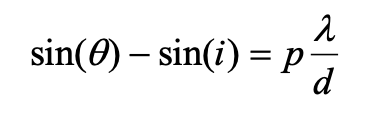p is the integer diffraction order.

The relation giving the diffracted intensity for small angles (i~θ):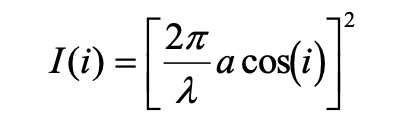for a depth a of the grooves.

Of course, one cannot go very far with this treatment. But on top of being very easy to grasp, it already shows characteristic features which will stay with us in the most advanced theories of surface scattering.

• The correct independent angular variable is: sin(θ) - sin(i) which the specialists of scatterometry usually write : β s - β i .
• If you change the incidence angle, the scattered intensity drops as the square of the cosine of the angle.

The exact vectorial theory

Considerable amount of work has been devoted to surface scattering. Finally, the most exact treatment is known as the Rayleigh-Rice perturbation theory.

This yields the following result :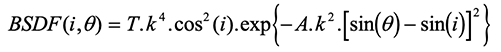where T is the transmission of the sample and k = 2π/λ.

This equation is somewhat simplified :

• it is written for small angles and projected in the scattering plane
• it does not account for polarization effects

It is remarkable that we find again the two features we had pointed out from the grating theory. A third useful feature is comprised in the Rayleigh-Rice relation: the fourth power dependance on wavelength. It can be used to compute the BSDF at a wavelength from the measurements made at another wavelength.

Another important result that can be drawn from this theory is the relation between the µ-roughness of the surface and the total scattering of this surface.

Definition :

If the surface profile is z(x) measured at N equidistant points x i along along a line of length L with respect to a reference chosen such that Σz(x i ) averages to 0 over L, the rms µ-roughness δ is defined as :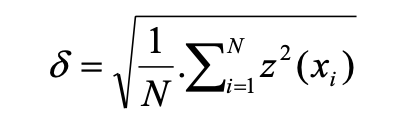The Total Integrated Scattering ( TIS ) is defined as the ratio of the total scattered power over 2π by the total reflected power.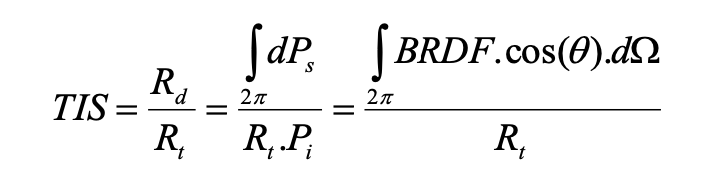One deduces from the vectorial model :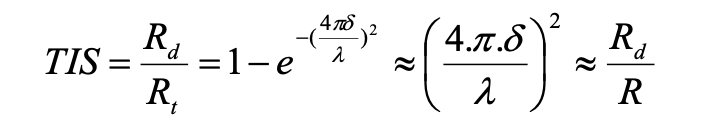The approximation holds for 4.π.δ<<λ which is also the condition for the theory to be senseful. In this case the total reflectivity and the specular reflectivity are very close together and one can use the specular reflectivity easier to measure; but one should keep in mind that this is invalid as soon as scattering exceeds a fraction of 1%, i.e. as soon as we deal with a dirty mirror.

The following table shows numerical values for a wavelength of 650 nm and various ratios δ/λ.

One sees that for a µ-roughness of 40 nm, the TIS is 85% and half the light is lost due to scattering; normal astronomical mirrors must have a µ-roughness of 5 nm (bad mirror) down to 0.5 nm very smooth mirror or plate used for coronography.

Dust or pollution scattering has nothing to do with the Raleigh theory and cannot be described in terms of µ-roughness.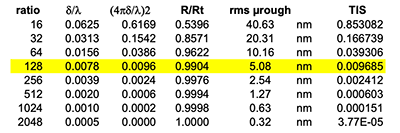Normal astronomical mirrors exhibit a mirror scattering loss ranging from 0.1% up to 1% at most if they have been properly commissioned.

A dirty or scratched mirror will exhibit a value of TIS and a related value of scattering loss that can be described by R/R t or R d /R; what makes no sense in this case is the µ-roughness value which is scientifically wrong and gives no feeling whatsoever of the damage to good observation due to the state of the mirror, while R d /R gives an immediate grasp of what is going on.

The empirical Harvey relation

As we already pointed out, this is in fact all we need in order to build the most economic and reliable instrument. The Harvey model of surface scatterer follows the law :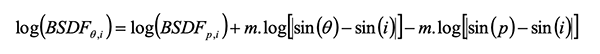Where b is a conventional “pivot” angle, usually very small, and m is the slope of the log-log diagram of the BSDF as a function of the angular variable |sin(θ)-sin(i)| or |β si | . The Harvey relation is mostly utilized in this logarithmic form because its structure is very simple (a straight line of slope m):

Y = Y 0 + m*X

where Y = log(BSDF), X = log(|β si |) and Y 0 comprises the constant terms.

One notice that if two BSDF measurements are performed on a sample at two different angles, the Harvey relation allows to compute b = BSDF p,i and the slope m . The choice of p is arbitrary and can be chosen as a significant angle for the particular application one has in mind. A common choice is i-.0573° because then β s = .01 which is easy enough to keep in mind. For IRIS , CT7 and CT7a , we have chosen p = 45°-1.75° and the instrument will compute b and m from the measurements at 45° and at 15°. These data then allow to compute the BSDF at any angle.

The Harvey relation turns out to be an excellent representation of the scattering behaviour of well-behaved surface scatterers such as clean polished mirrors with small defects. If the scatterer is not well behaved (as a dusty mirror for instance), the Harvey law still represents good portions of the angular dependency of the BSDF , but is less accurate for angles outside the range of the two calibration angles.

Application of the Harvey relation

Some typical Harvey curves are reproduced hereafter. One sees that, for normal polished glass, the Harvey relation is quite accurate in a very wide angular domain. The cyan curve is for a not well behaved scatterer as a narrow angle diffuser. The smallest scattering angle is 15 arcmin and the two arrows show the Iris / CT7 / CT7a measuring angles.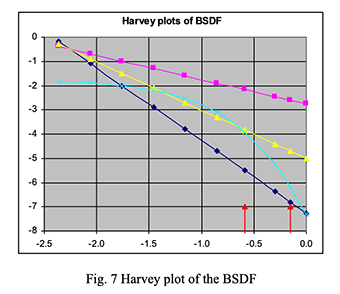A typical astronomy application would be the following. Assume that you measure a faint stellar-like object close to a bright star with a pixel detector; that the pixel size is .5"*.5", the seeing is .3". The bright object is mag 2; what amount of (parasitic) light from the bright object is polluting your faint object if the distance between the two object is 5" and the Iris / CT7 / CT7a scattering measurement on the primary yields the values m=-2.168 and b=.3618 ? Assume no scattering at mirrors other than primary and normal incidence on primary (i = 0).

One finds the scattered power at angle θ from specular (taken as normal incidence for simplicity):

δP s = BRDF(0,θ).P i .δΩ

which in our example evaluates to :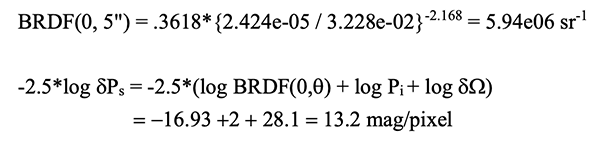Limitations of the Harvey relation : the ABg system.

The main drawback of the Harvey representation is that it breaks down at very small angles since its mathematical formulation exhibits a singularity at θ = i .

The Harvey relation in non-logarithmic form reads :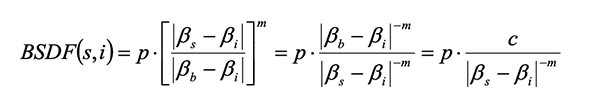where m is a negative number with a value usually comprised between –1 and -3, so that for s=i the expression yields an infinite value.

This makes the result of the preceding section approximate, since the BRDF at .5" has been deduced from measurements made at 15° and 45°: the result should not be used in correcting the photometry of the faint object, but just to make a preliminary estimate of what can be expected. For instance, such computation will show immediately whether the mirror cleanliness is critical for a given observation. The actual photometric calibration of the scattering should be performed by measuring an empty field at the same distance of the bright star.

The Harvey model has been modified in order to remove this singularity. This new model is known as the ABg or Harvey-Shack model; it is defined by the relation: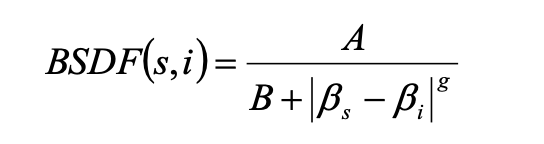Unfortunately, if you measure the BSDF for three angles s 1 , s 2 , and s 3 , there is no way you can compute the corresponding A, B and g coefficient algebraically. That is why Iris / CT7 / CT7a computes the Harvey coefficients b and m which can be computed algebraically (c=.0232 m is known since the angle p has been chosen to be 43°.25 and i=45°).

While the log-log plot of the Harvey relation is a straight line with slope –m, the ABg log-log plot flattens out for small angles (less than 1° or at most 2° from the specular direction). This roll-off takes place for scattering angles such that B=(β rolloffi ) g . Typical roll off angles for normal incidence and g=1, 2 and 3 common values are given in the following table :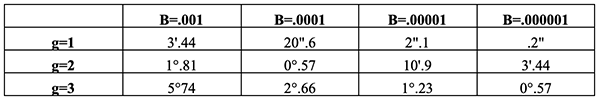For β s <<β rolloff , BSDF =A/B and is constant, while for angles greater than a few degrees, the BSDF assumes the Harvey shape (straight line with slope m in log-log plot). Measuring the BSDF at an angle β srolloff is the only way to determine the parameter B of the ABg system. In view of the smallness of β s the best way to perform this measurement would be to measure the scattered light around a bright object in the focal plane of the telescope, where a resolution of the order of one arcmin is easily feasible. An approximate way is to measure the scattering at a small angle (for instance 2°), compute the A and g parameters from the Harvey relation using measurements at large angles (15° and 45° for Iris , CT7 and CT7a ) and fitting the value of the measurement at 2° to determine the value of the B coefficient of the Harvey-Schack model. This is what we do in the new version of Iris , CT7 and CT7a .

The ABg system is interesting if we want to estimate the diffusion at 1" to 10" from a bright object as in our example. Here, we deal with values of β s g in the 10 -9 to 10 -10 range. In this range, the stray light from the mirror is always flat with a value A/B and will have to compete with the tail distribution of the seeing.

If the mirror is dirty, the value of A and B are larger. The following diagram shows the fit of ABg curves to measured BRDF samples.

ES is a clean aluminized mirror : Fp is the same with a plain finger print; Mean is a glass with µgrit 12 scratches; Bad is the same but badly damaged; Calc is a glass with conspicuous traces of calcium due to repeated water evaporation. One can see that the ABg formalism can represent accurately the scattering data. The ABg parameters and the TIS are given in the following table.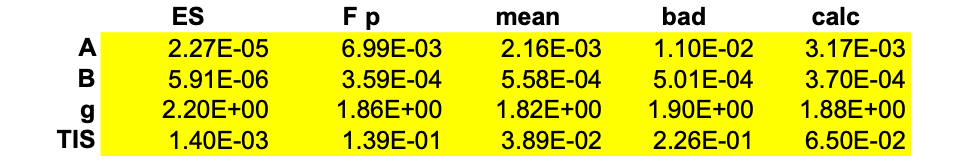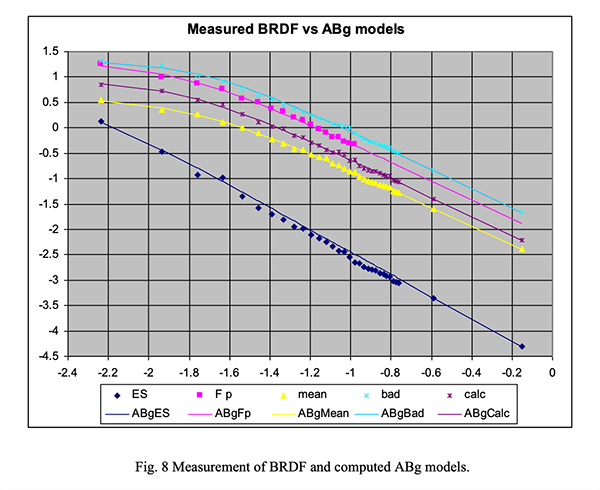One sees that a small value of A indicates a low global scattering, a low value of B indicates a clean mirror scattering at small angles; a large value of B indicates a dirty or scratched mirror scattering at large angles; the g exponent has generally a value around 2 : larger for a clean mirror, somewhat lower for a dirty one.

One word of caution is necessary if you want to perform quantitative measurements and computations using the BRDF and the TIS . The TIS computed by ray tracing programs (for instance Zemax) defines the TIS without the reflectivity term, that is :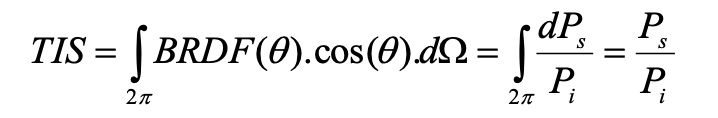It has the advantage of being defined dependently of the reflectivity of the surface.

The TIS defined previously following the text books goes to unity for a pure scatterer (specular reflectivity is zero). It can be measured directly on a sample using a Coblentz sphere as shown hereunder. The sphere is optically polished and has a known reflectivity R cob ; symmetrically with respect to the center of the sphere C and close to it are placed the sample S and a detector D; the incident beam I is directed through a small hole in the sphere towards the sample; the specularly reflected beam leaves the sphere through another hole and is measured by a detector R. The scattered light appears optically as a small source on the sample and emitting light in the whole hemisphere; from the Snell’s laws, it is imaged on a small surface symmetrically positioned with respect to the center of the sphere, where it is captured by the detector D.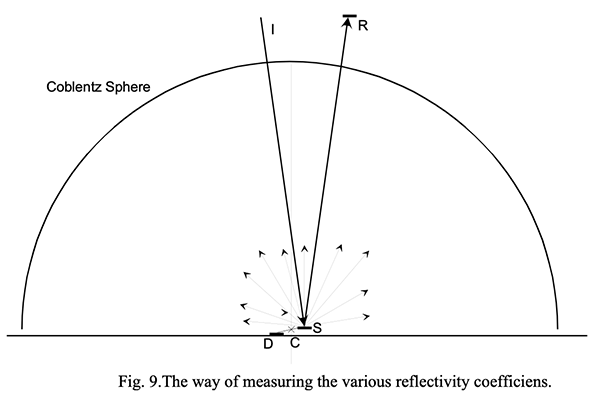In this setup, The detector R is positioned alternately to measure P r (the specularly reflected power) and P i , (the total incident power); The detector D measures either P s the purely scattered power if the exit hole for the reflected beam is open, and Pt, the total reflected power if the hole is closed by a reflective plug. From these measurements, one can deduce all the quantities that we have just defined in both systems as it is shown in the table below.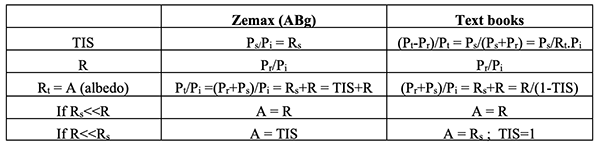It is very important to be noted that the only reflectivity that is meaningful for astronomers is the specular reflectivity R; only the light reflected at the specular angle forms an image; the rest is wasteful and increases the background. Consider the next figure: it is a plot of the total reflectivity at various angles (the x scale is the radius of the detector with the star image at the center). It uses the text books definitions.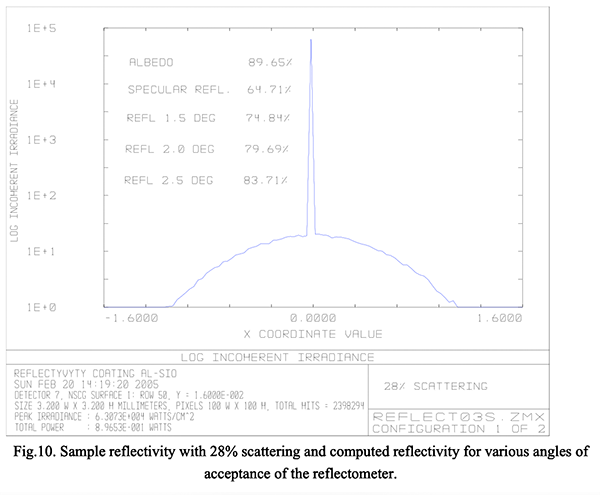This is a sample mirror with very heavy scattering (28%) the various reflectivities have been entered and one can read : A= 89.65% and R=64.71% : this is just the power in the central peak of the figure divided by the incident power, measured with a very small detector (1 arcmin); this power is not dependent of the radius of the detector between 10 arcsec and about 10 arcmin. When increasing the size of the detector further, scattered power progressively adds its contribution to the specular power and the apparent reflectivity increases; figures are given for three detectors of current acceptance angle: 1.5°, 2.0° and 2.5°. One sees that the error on ‘reflectivity’ measurements is quite substantial and it really makes no sense to measure directly the reflectivity of a sample with heavy scattering; the measure depends much on the exact value of the acceptance angle of the instrument and will differ from one instrument to the other.

The Beekman theory

The beekman theory is a trial for obtaining a theory of scattered light when λ << σ.

For normal incidence, the following relation is given :

BRDF(s) = π*R*e -[sin(s)/(2S)/(1+cos(s))]^2 *{2πS*(1+cos(s))} -2

Where s is the scattering angle, R is the specular reflectivity and S=σ/Λ the ratio between the rms µ-roughness and the autocorrelation length, which is a measure of the average slope of the surface accidents.

The following plot compares the ABg curve to the Beekman result, for a common value of scattering at small angles : one should notice that the Beekman relation having only one free parameter, when using this parameter to adjust the scattering at small angles, the rest of the curve is entirely determined. The value of S used is 0.118, and the corresponding Abg values are respectively .00028; .001; 2. The angles range from 0.17 arcm (-4.31) up to 71° (-.02); the roll of point of the Abg curve is around 30 arcm (-2.06),and of the Beekman curve around 8° (-0.86). It is apparent that apart for the common value of the scattering at very small angles, the two curve bear no resemblance at all.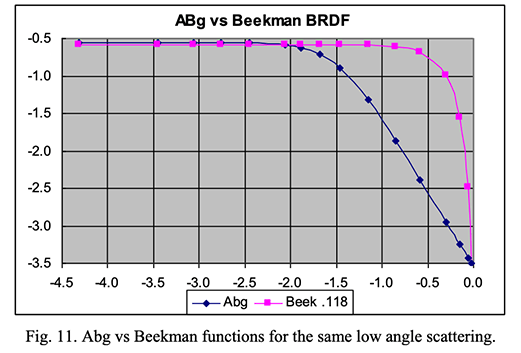The Beekman curve has not been observed nowhere; from our discussion at the beginning of this note (what causes optical scattering?), this failure of a “theory” of scattering for σ>>λ is not surprising.

The Gauss scattering function

A very simple way of representing a scattering function is to use a Gauss distribution; this procedure has the merit of being easy and fast to introduce in ray tracing computations.

The Gaussian scattering function is defined by :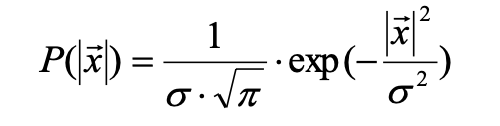where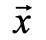is the projection on the scattering surface of the difference between the scattering unit vector and the specular unit vector (so that, if the icidence angle is 0°,is the sine of the scattering angle. P is the total power scattered for a given value of, the azimuthal distribution being uniform), and σ is the width of the distribution (a Lambertian distribution has σ > 4; most dust scatterers have 0.25<σ<1). The Gauss distribution is however far from a Harvey distribution: it has no range of the angular variable with a constant slope so that it is too flat for small angles and too steep for large angles. It is useful when considering a very limited angular range and when estimating scattering due to mounts in optical instruments.

### What measurements are important for telescope mirrors maintenance?

This is of course the final question; the answer depends somewhat on the usage of the telescope and the conditions in which it is used.

If you want to do spectroscopy of faint stars, scattering by the mirrors and even by the atmosphere is not so detrimental as if you run after direct detection of planets around stars. But of course, a large telescope has to provide the required service to a large community of astronomers and since you cannot switch the mirror’s coatings from poor to excellent depending on the program of the night, the coating should always be ready for the best performance. The most reasonable attitude is to take into account that there will necessarily be some decay of the coatings performance between two maintenances and to arrange the program following the planned maintenance: The most demanding programs should be scheduled soon after maintenance, and the less demanding towards the end of the maintenance period. This of course supposes that the maintenance is carefully planned and that the mirrors have been monitored so that a good prediction of their state is possible.

As we have seen from the discussion of the BRDF, the only part of scattering interfering with astronomical observations is the scattering at small angles (say less than 15arcm); scattering within this range is determined by the microroughness of the mirror, but is way out of the range of any measuring scatterometer; the only sensible way to measure it accurately is to measure it directly in the focal plane of the telescope (certain handy scatterometers yield a measurement of the microroughness; this is based on higher order scattering and is not very accurate; it should be noted that as soon as there is the least trace of dust or pollution on the mirror, the high order scattering due to microroughness is completely masked by the direct scattering of the pollutant; the scatterometer will of course still indicate a value for the microroughness, but this value has no signification whatsoever : it is an arbitrary figure that shoud not be utilized for pollution monitoring). The scattering at larger angles (say larger than 2°) is mainly due to dust and pollution; it can be measured by a scatterometer and provides a good estimate of the cleanliness of the mirror. However, it is little related to the performance of the mirror, because, unless the pollution is massive, scattering by dust has no effect on the resolving power of the telescope and very little on the specular reflectivity. Note that the scattering at large angles is not related to the microroughness unless the mirror is absolutely clean. Hence, the microroughness value delivered by a scatterometer is usually quite arbitrary. For estimating the cleanliness of a mirror one should exclusively use the BRDF at various angles or the Harvey coefficients (p and m), or the ABg coefficients of the Harvey-Shack distribution.

The reflectivity is of course the main parameter which is of interest, but one should understand the specular reflectivity . As an astronomical instrument is always near diffraction limited, large telescopes are limited in the first place by atmospheric seeing and atmospheric scattering. Seeing can be partly corrected, but not atmospheric scattering. The latter takes place in the atmosphere and nothing can be done against it; it is especially detrimental when the full moon is in the sky. In this paper, we are dealing with the scattering by the mirrors of the telescope itself. The reflectometer has necessarily an acceptance angle; the acceptance angle of conventional reflectometers is 2° (narrow) or 10° (wide); in good instruments, this angle is limited and is somewhat lower than 2°. But anyhow, this angle ( Iris , CT7 and CT7a have 1.5°) is still too large for measuring accurately the specular reflectivity if the total scattering exceeds a few percent; what is measured by an instrument with an acceptance angle larger than 0.2° is a mixture of specular reflectivity and scattering. With an acceptance angle of the order of 10° the result of measurements is closer to what astronomers call the albedo, that is the sum of the Total Integrated Scattering ( TIS ) and of the specular reflectivity (which forms a sharp image). Using a narrow acceptance instrument (1.5° to 2°), the result is closer to the correct specular reflectivity when the scattering of the mirror is lower, and closer to the albedo when the scattering is large. This is why it is necessary to measure both ‘ reflectivity ’ and scattering when monitoring a telescope mirror.

For instance, if we consider a pure aluminum coated mirror with various total integrated scattering ( TIS ), and specular reflectivity R, ray tracing will yield the results shown in the following table for measurements with reflectometers of various acceptance angles.

The first column is the specular reflection (the true measurement one wants to obtain); the last column is the albedo or total reflectivity (including scattered light) that would be measured by a MINOLTA albedo meter for instance. Different diffusing functions have been examined: Gauss 2.0 is approaching a Lambertian diffuser, very unlikely to represent a dusty mirror; Gauss .5 is probably the most representative of an actual mirror situation. For each BRDF, three degrees of dirtiness have been computed: a relatively clean mirror (1% total scattering), a relatively dirty mirror (10% total scattering) and an intermediate case. One sees that the specular reflectivity differs from the albedo by the quantity of scattered light which is simply subtracted from the albedo in obtaining the specular reflectivity . The second important point is that for clean or moderately dusty mirrors, the measurements performed with instruments having an acceptance angle between one and two degrees are quite accurate: they yield a measurement which is at most 0.28% to high. If the mirror is quite dirty, the difference goes up to nearly 1%; for samples where the TIS reaches values in the range of 20% to 50%, the differences would reach between 5% and 10%.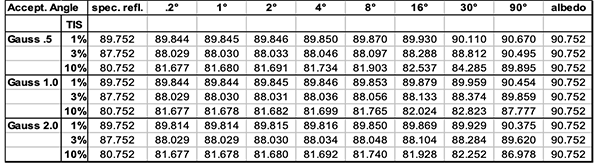Practically however, I cannot imagine that a telescope mirror would be allowed to become so dirty as to show more than 5% scattering and therefore one can use a narrow angle reflectometer, using a scatterometer to get a feeling of how accurate the reflectivity measurement is, that is how close it is to the specular reflectivity.

However, the total scattering is not easy to measure accurately with a portable instrument. One can estimate its value by measuring the scattering at one or, better, two angles assuming a model for the scattering function. This is a good practice, because the scatterer is always the same at a given location, although it might differ from one place to the other. We now turn to the task of estimating the TIS from the measurements at three angles. We have seen by examining the actual ABg curves measured on various samples that the maximum angle where the curves differ from the Harvey straight line is between 1° and 2° depending on the cleanliness of the mirror. On the other hand, one will see when examining the measurement technique that the minimum angle where scattering can be measured is around 2°. This angle is then the only choice we have for the time being. Let us examine what it means for the instrument and what difficulties such a small angle brings.

The general structure is shown on the drawing : the source sends a laser focused beam on the sample at an angle of 45°; the detectors at -15° and -45° are not represented : they bring no difficulties. The detector at 2° should discriminate between the specular beam and the scattered beam at 2° from the former. The difficulty stems from the fact that for relatively clean mirrors, the power in the specular beam is 10 4 to 10 5 times the power in the scattered beam.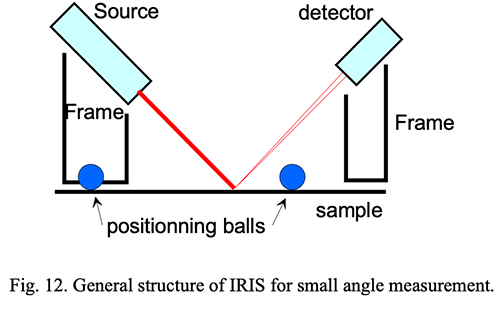The frame and positioning balls are sketched on the figure; these are important elements for maintaining precisely the angle relations between the beams. A three-points contact is always a perfect positioner on any surface; even with a parabola, the angular difference at the incidence point is much smaller than 1° and the return beam to the detector will always be correctly positioned. This would not necessarily be the case if the positioning interface were an o-ring for instance.

First of all let us examine the source because light scattered at 2° from the specular image should be dimmed by a factor of the order of 10 -6 , otherwise it will be measured as sample scattering.

The source comprises of a laser diode, a lens and baffling; the latter is the most important part for controlling stray light. First, the laser diode has an emitting junction which is very small (1x5µm) and can be seen as a point source. But it emits light on both sides and part of this light is scattered and reflected around by metallic parts and by the output window; finally the point source is surrounded by a diffuse source of the size of the window; this is maybe 10 4 times dimmer than the point source but in measuring scattering it is much too high; it is attenuated by the first baffle just against the diode with a 0.3 mm pinhole.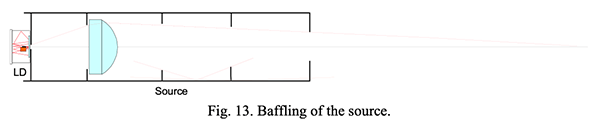Then the lens itself is completely bright if AR coating is not very efficient (no coating means a large source with a total brightness of the order of (0.04*0.04 = 0.0016) times the point source. Then the other baffling builds up a series of black boxes killing grazing incidence high reflectivity on the tube (lower part of the figure). Finally, this source configuration has been abandoned because it turned out impossible to reduce the projection on the detector of the scattering inside the laser diode window and maintain the laser stability. In the final configuration, we use a pigtailed deep blue diode and define the parallel beam by two pin holes just large enough to avoid diffraction. Intermediate larger diaphragms take care of grazing incidence internal reflections.

Let us now turn to the detector cavity. Light is entering from the right through the 2.5 mm opening of the first stop; the total length of the cavity is 19 mm.

The first three small cavities (1; 2; 3) separated by stops are there to trap the stray light and prevent grazing incidence reflections. The last cavity is where the specular beam is discriminated from the scattered beam at 2° (dotted lines). The first stop (4) of this cavity is shown seen from the front on top of the drawing; it has one central hole 1 mm diameter surrounded by sixteen 0.3 mm holes on a radius if 1.1 mm corresponding to 2°. These holes are fit with silica fibers of 0.225 mm core which conduct the light through the second stop (5) to the detector. The holes in stops 4 and 5 are completely obscured around the fibers as well as the inside of the last cavity by a special black epoxy. The specular beam (not represented on the figure) is completely trapped in a conical cavity behind the 1 mm stop in between the fibers. A photograph of the two stops and fibers of the last cavity is shown hereunder.